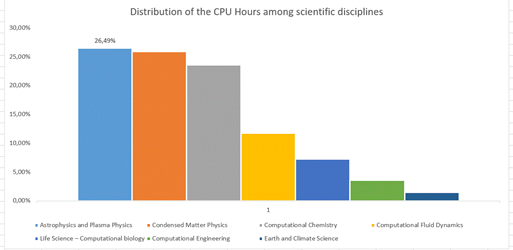# ISCRA - Statistics

ISCRA CLASS B CALLS

CALL XVIII (2018/2)

 Total Number of proposals presented 63 Total Number of cpu hours requested 70.000.000 (A1) + 200.000.000 (A2) Accepted proposals 42  (67%) Cpu hours assigned 40.000.000 (A1) + 128.000.000 (A2) Numbers of independent referees involved 70CALL IXX (2019/1)

 Total Number of proposals presented 42 Total Number of cpu hours requested 40.000.000 (A1) + 200.000.000 (A2) Accepted proposals 32  (67%) Cpu hours assigned 18.000.000 (A1) + 120.000.000 (A2) Numbers of independent referees involved 84